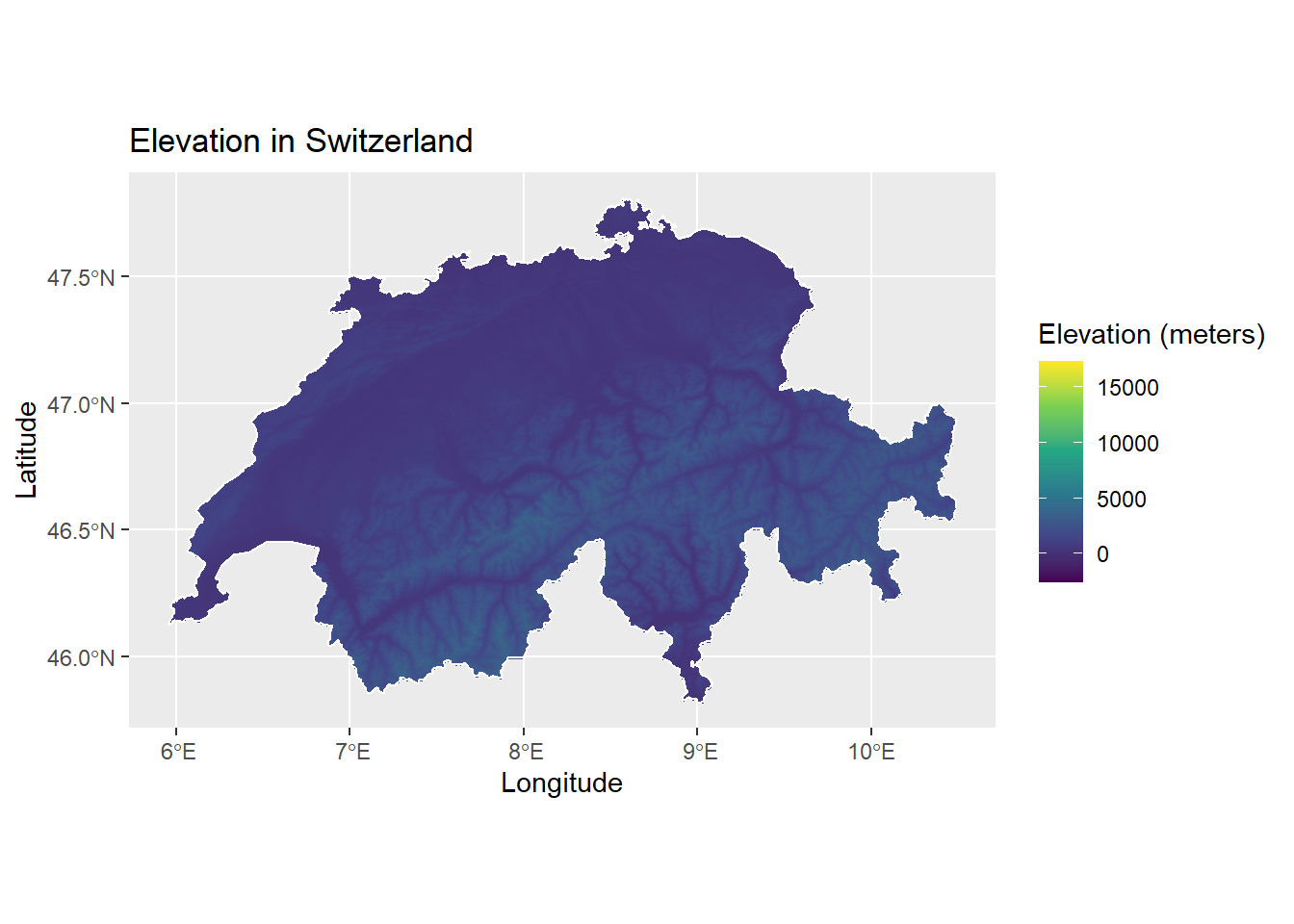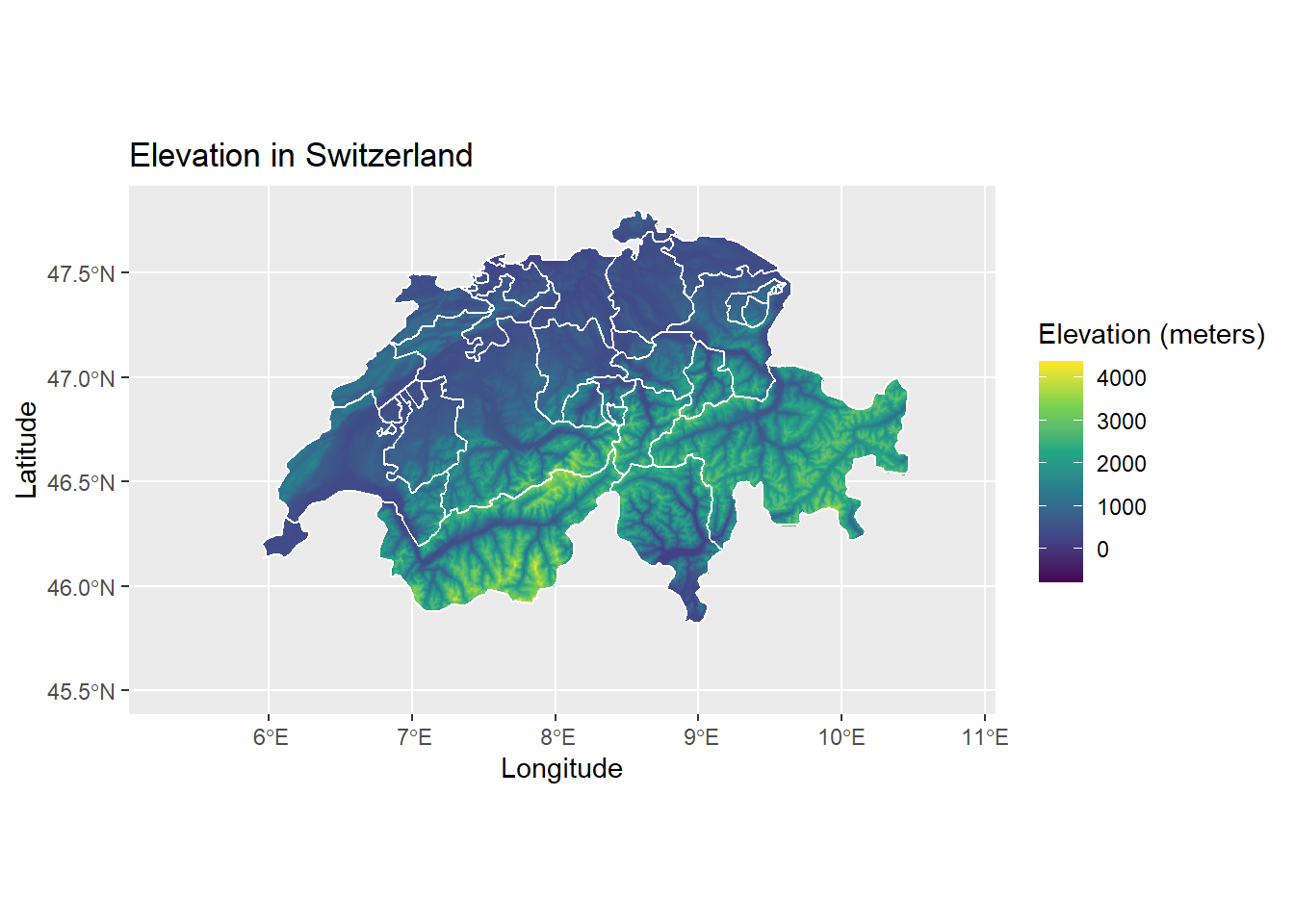#### Data set details

 Data set description: Elevation data in Switzerland Source: USGS Elevation Point Query Service and Amazon Web Services Terrain Tiles Details on the retrieved data: Elevation in Switzerland along with the data on the administrative areas for the same country. Spatial and temporal resolution: Elevation data with different zoom levels (see details)

# Downloading elevation data with the elevatr package

This tutorial gives you a brief understanding of how to use the elevatr package for a standardized access to the elevation data from the web. The packages uses two endpoints to access its data from:

## Installing the package

The elevtr package can be directly downloaded from CRAN as follows.

install.packages("elevatr")

Loading the elevatr package:

library(elevatr)

## Functions available

Currently, there are two functions in this package which help users access elevation web services, namely, get_elev_point() and get_elev_raster().

The get_elev_point() function gets the point elevations using the USGS Elevation Point Query Services (for United states only) and AWS Terrain Tiles (for all global elevation data).

• Input: This function accepts a data frame of longitude and latitude values (x and y), a SpatialPoints/SpatialPointsDataFrame, or a simple feature object (sf). It has a source argument src which indicates which API to use, either "eqps" or "aws".
• Output: produces either a SpatialPointsDataFrame or Simple Feature object, depending on the class of input locations.

The get_elev_point() function can be used as follows:

ll_proj <- "+proj=longlat +ellps=WGS84 +datum=WGS84 +no_defs"
elev <- get_elev_point(pt_df, prj = ll_proj)

library(kableExtra)
elev %>%
kable() %>%
kable_styling(bootstrap_options = c("striped", "hover"))
elevation elev_units coords.x1 coords.x2
NA meters -71.82791 40.25963
243.79 meters -71.76843 43.05586
73.74 meters -71.37858 42.52338
86.83 meters -71.10417 42.65332
391.93 meters -71.56750 43.91346

The get_elev_raster() function helps users get elevation data as a raster from the AWS Open Data Terrain Tiles. The source data are global and also contain the estimations for depth for oceans.

• Input: This takes in a data frame of longitude and latitude values (x and y) or any sp or raster object. It has a z argument to determine the zoom or resolution of the raster (1 to 14). It also has a clip argument to determine clipping of returned DEM. Options are "tile" which is the default value and returns the full tiles, "bbox" which returns the DEM clipped to the bounding box of the original locations, or "locations" if the spatial data in the input locations should be used to clip the DEM.

• Output: Returns a raster object of the elevation tiles that cover the bounding box of the input spatial data.

We can use the get_elev_raster() function to obtain the elevation data of Switzerland, and plot it together with the country boundaries obtained by using the rgeoboundaries package as follows.

# remotes::install_github("wmgeolab/rgeoboundaries")
# install.packages(c("ggplot2", "raster", "elevatr", "sf", "viridis"))
library(ggplot2)
library(raster)
library(elevatr)
library(rgeoboundaries)
library(sf)
library(viridis)

swiss_bound <- geoboundaries("Switzerland")
elevation_data <- get_elev_raster(locations = swiss_bound, z = 9, clip = "locations")

elevation_data <- as.data.frame(elevation_data, xy = TRUE)
colnames(elevation_data) <- "elevation"
# remove rows of data frame with one or more NA's,using complete.cases
elevation_data <- elevation_data[complete.cases(elevation_data), ]

ggplot() +
geom_raster(data = elevation_data, aes(x = x, y = y, fill = elevation)) +
geom_sf(data = swiss_bound, color = "white", fill = NA) +
coord_sf() +
scale_fill_viridis_c() +
labs(title = "Elevation in Switzerland", x = "Longitude", y = "Latitude", fill = "Elevation (meters)")We can also get the different administrative areas of the country Switzerland, by using the ne_states function from the rnaturalearth package. And the get_elev_raster function, you can get the elevation data. The following code does the same:

NOTE: increasing or decreasing the z argument will make the map zoom in and out on the country by setting the appropriate z-axis value.

# install.packages("rnaturlaearth")
# remotes::install_github("ropensci/rnaturalearthhires")

library(rnaturalearth)
library(rnaturalearthhires)

sf_swiss <- ne_states(country = "switzerland", returnclass = "sf")

elevation_1 <- elevatr::get_elev_raster(locations = sf_swiss, z = 7, clip = "locations")
cropped_elev <- crop(elevation_1, sf_swiss)
elevate <- as.data.frame(cropped_elev, xy = TRUE)

colnames(elevate) <- "elevation_value"
elevate <- elevate[complete.cases(elevate), ]

ggplot() +
# geom_sf(data = st_as_sfc(st_bbox(elevation_1)),color = "grey", fill = "grey",alpha = 0.05) +
geom_raster(data = elevate, aes(x = x, y = y, fill = elevation_value)) +
geom_sf(data = sf_swiss, color = "white", fill = NA) +
coord_sf(xlim = c(5.3, 10.8), ylim = c(45.5, 47.8)) +
scale_fill_viridis_c() +
labs(title = "Elevation in Switzerland", x = "Longitude", y = "Latitude", fill = "Elevation (meters)")